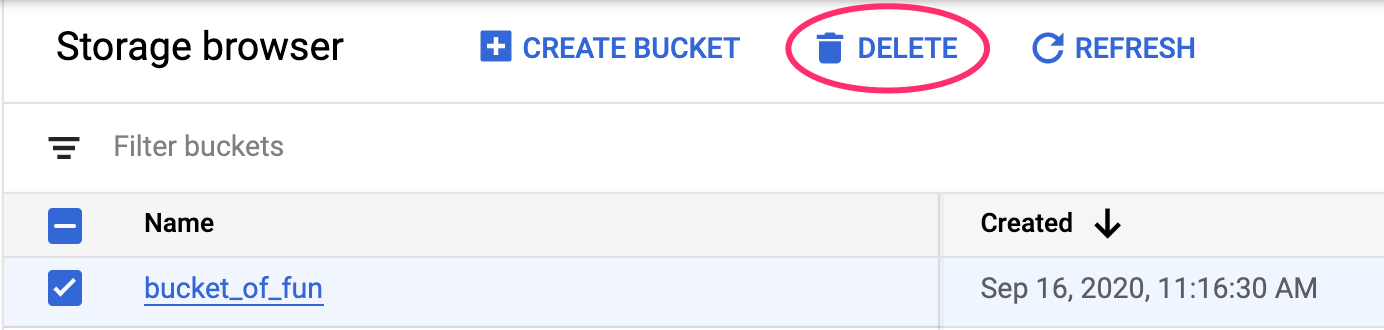# Get predictions from a pre-trained TensorFlow image model on Vertex AI

## 1. Overview

In this lab, you'll use Vertex AI get predictions from a pre-trained image classification model.

## What you learn

You'll learn how to:

• Import a TensorFlow model to the Vertex AI Model Registry
• Get online predictions
• Update a TensorFlow serving function

The total cost to run this lab on Google Cloud is about \$1.

## 2. Intro to Vertex AI

This lab uses the newest AI product offering available on Google Cloud. Vertex AI integrates the ML offerings across Google Cloud into a seamless development experience. Previously, models trained with AutoML and custom models were accessible via separate services. The new offering combines both into a single API, along with other new products. You can also migrate existing projects to Vertex AI.

Vertex AI includes many different products to support end-to-end ML workflows. This lab will focus on the products highlighted below: Predictions and Workbench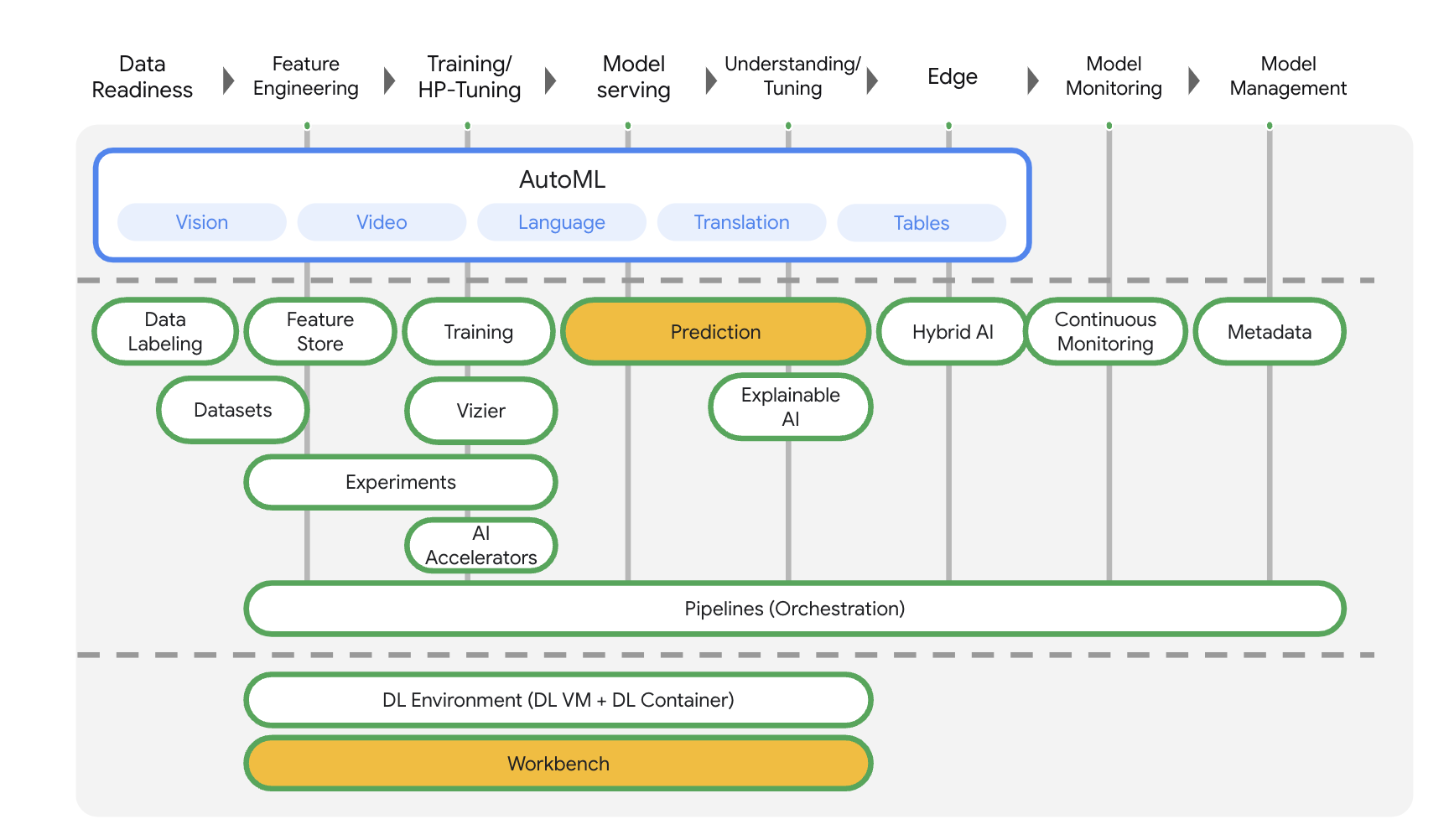## 3. Use case overview

In this lab, you'll learn how to take a pre-trained model from TensorFlow Hub and deploy it on Vertex AI. TensorFlow Hub is a repository of trained models for a variety of problem domains, such as embeddings, text generation, speech to text, image segmentation, and more.

The example used in this lab is a MobileNet V1 image classification model pre-trained on the ImageNet dataset. By leveraging off the shelf models from TensorFlow Hub or other similar deep learning repositories, you can deploy high quality ML models for a number of prediction tasks without having to worry about model training.

## 4. Set up your environment

You'll need a Google Cloud Platform project with billing enabled to run this codelab. To create a project, follow the instructions here.

## Step 1: Enable the Compute Engine API

Navigate to Compute Engine and select Enable if it isn't already enabled.

## Step 2: Enable the Vertex AI API

Navigate to the Vertex AI section of your Cloud Console and click Enable Vertex AI API.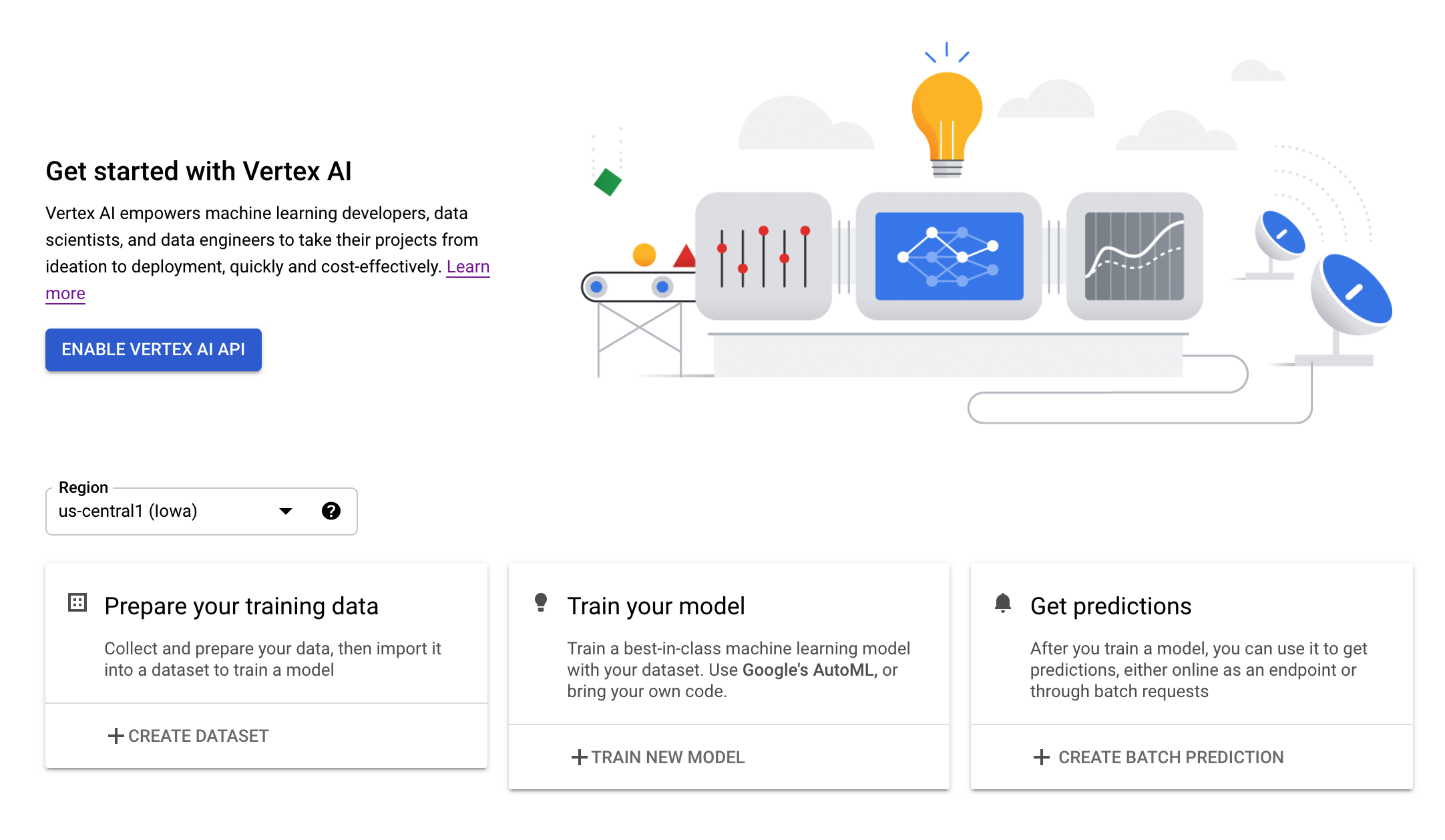## Step 3: Create a Vertex AI Workbench instance

From the Vertex AI section of your Cloud Console, click on Workbench: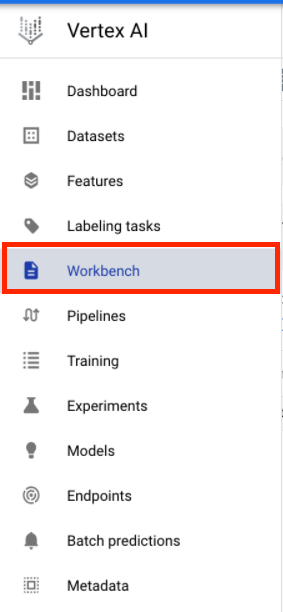Enable the Notebooks API if it isn't already.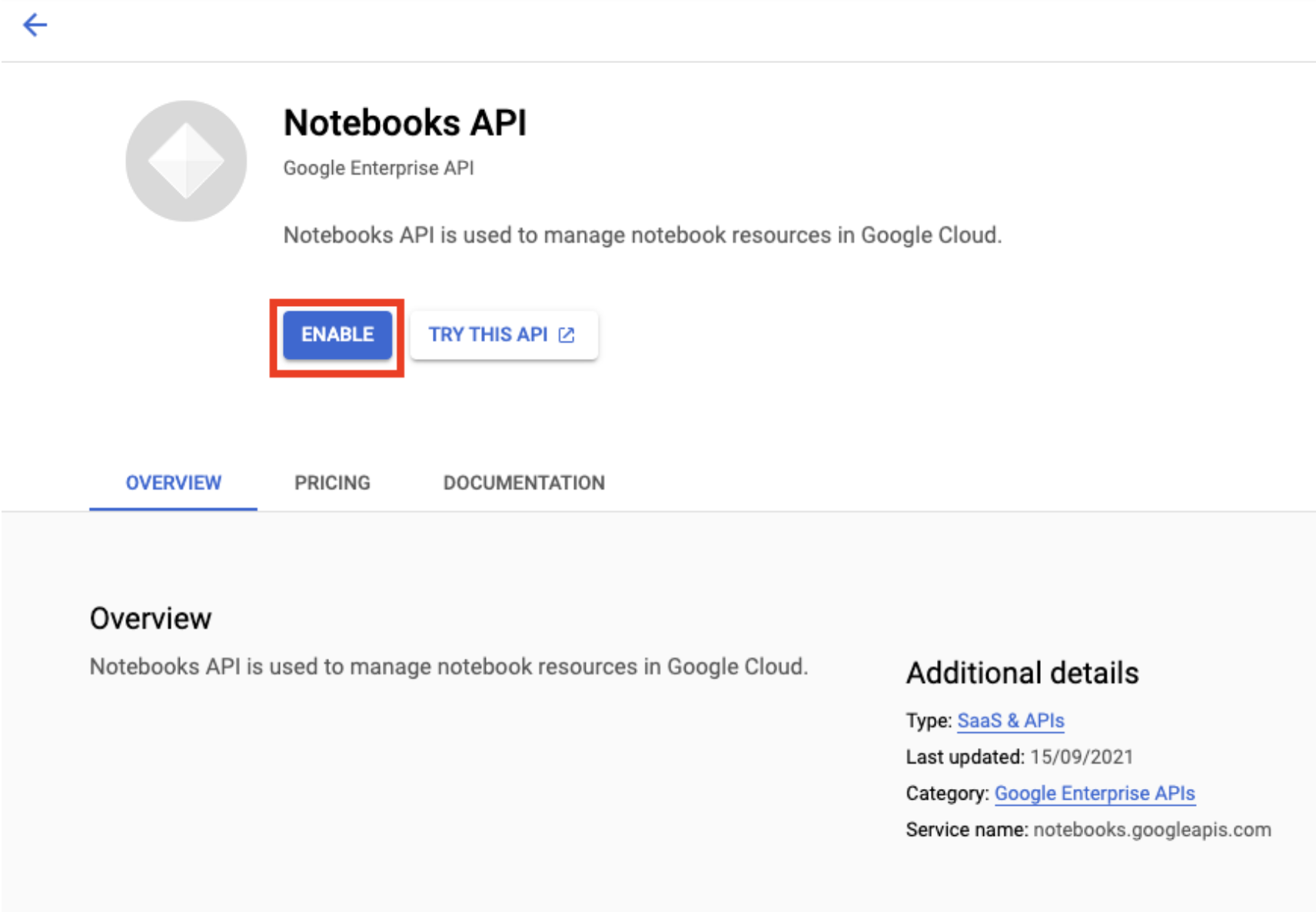Once enabled, click MANAGED NOTEBOOKS: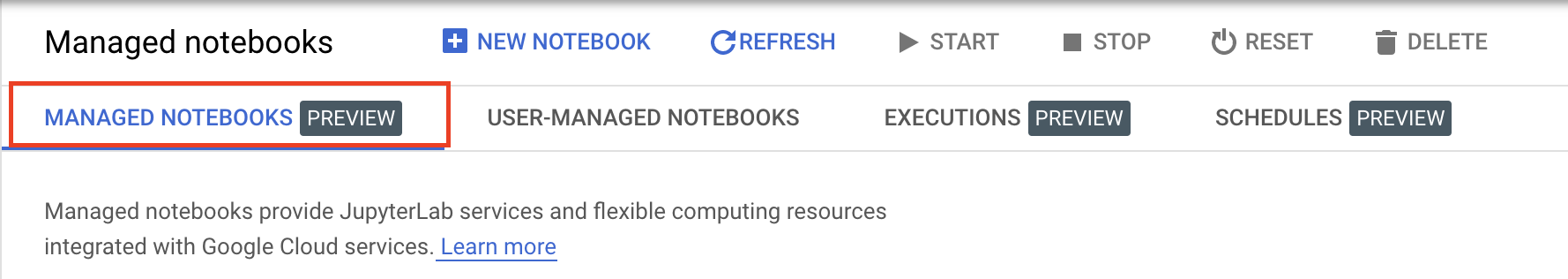Then select NEW NOTEBOOK.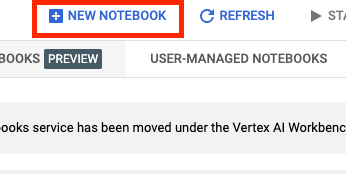Give your notebook a name, and under Permission select Service account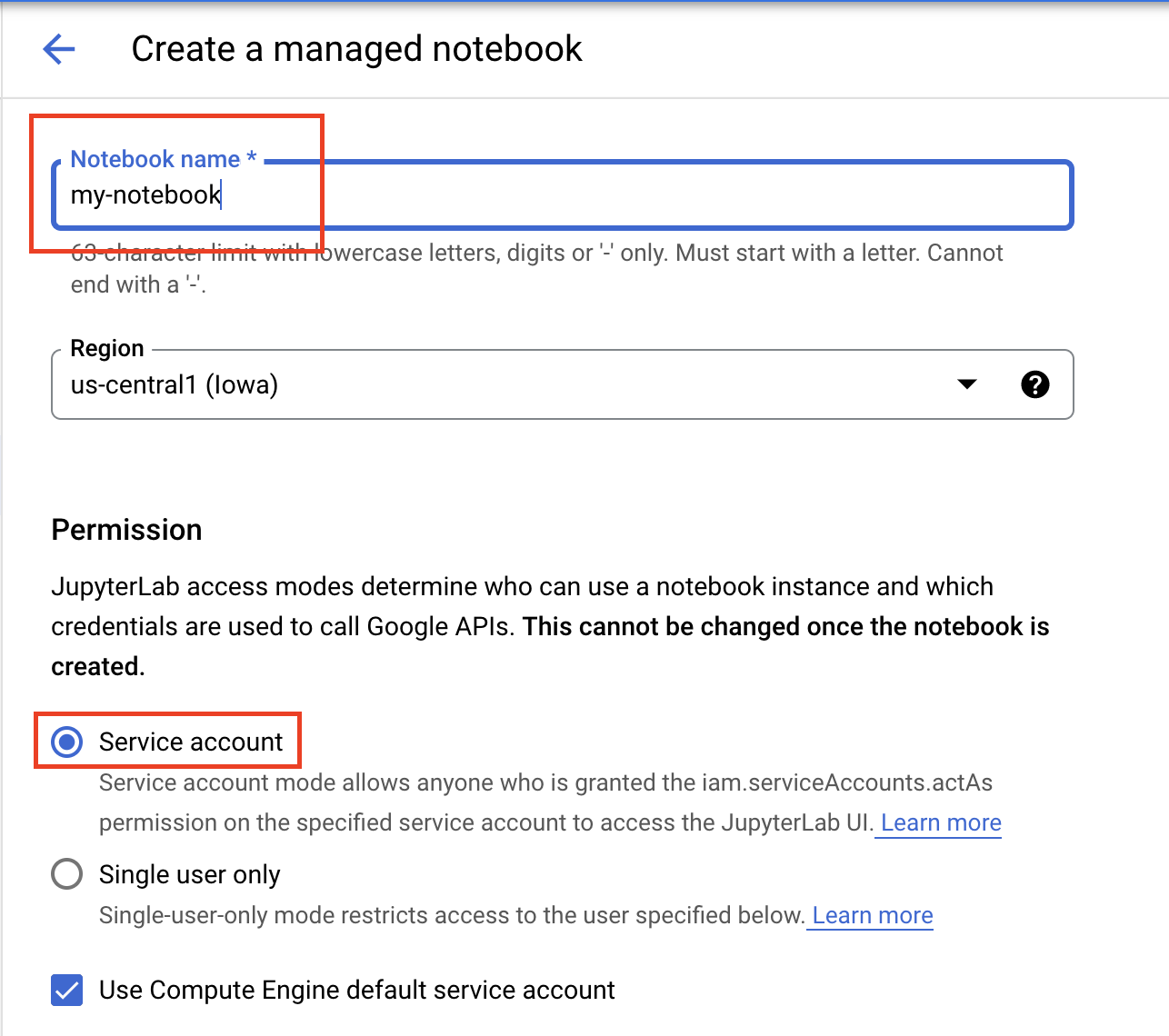Under Security select "Enable terminal" if it is not already enabled.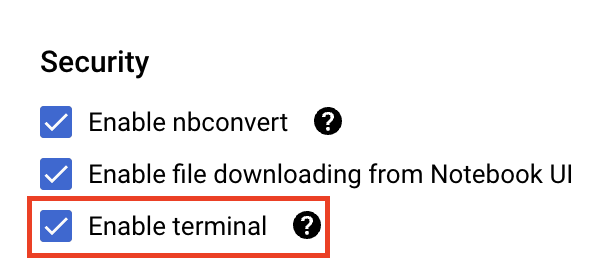You can leave all of the other advanced settings as is.

Next, click Create. The instance will take a couple minutes to be provisioned.

Once the instance has been created, select OPEN JUPYTERLAB.## Step 1: Upload model to Cloud Storage

Click this link to go to the TensorFlow Hub page for the MobileNet V1 model trained on the ImagNet dataset.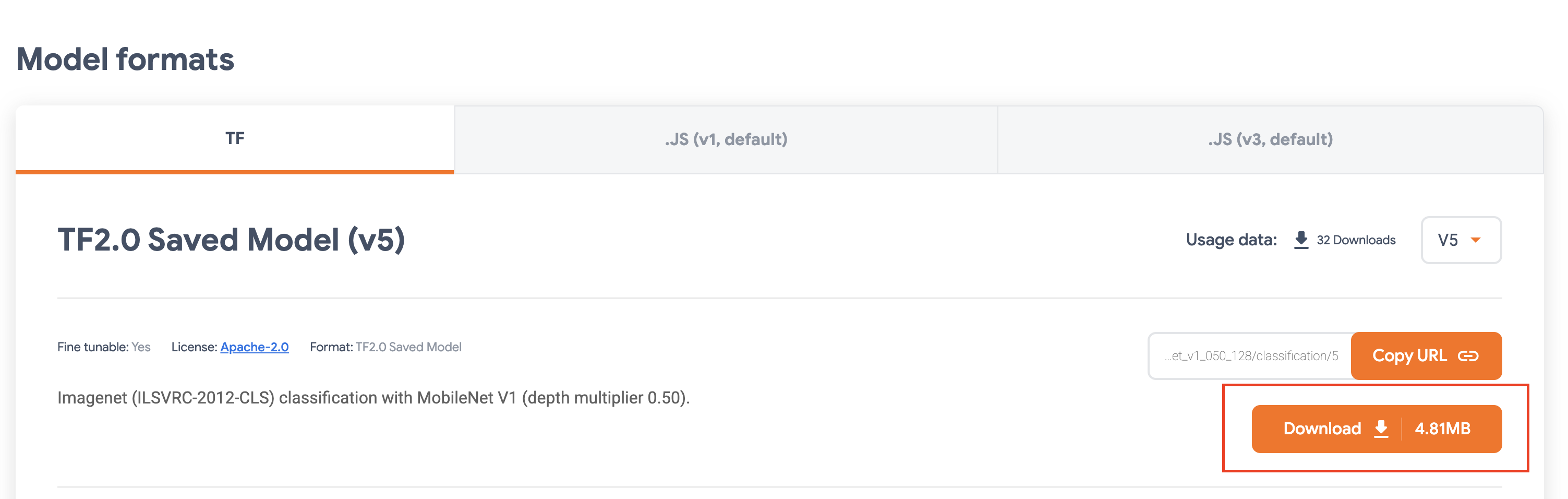From the Cloud Storage section of the Google Cloud console, select CREATE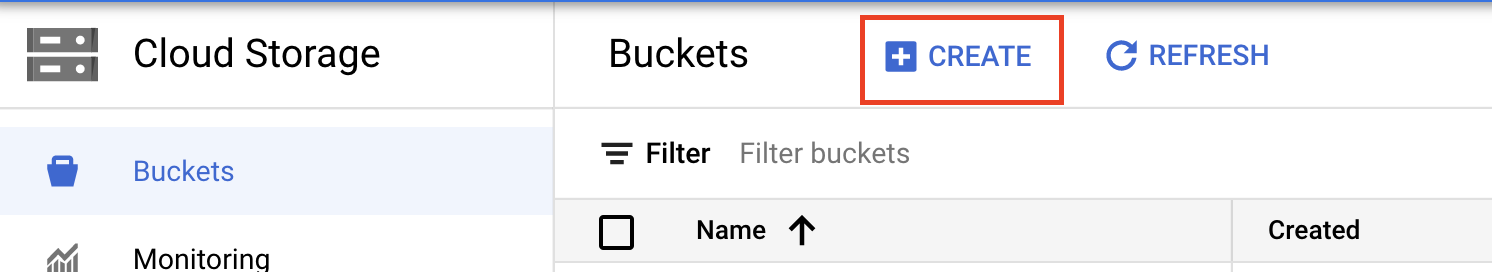Give your bucket a name and select us-central1 as the region. Then click CREATE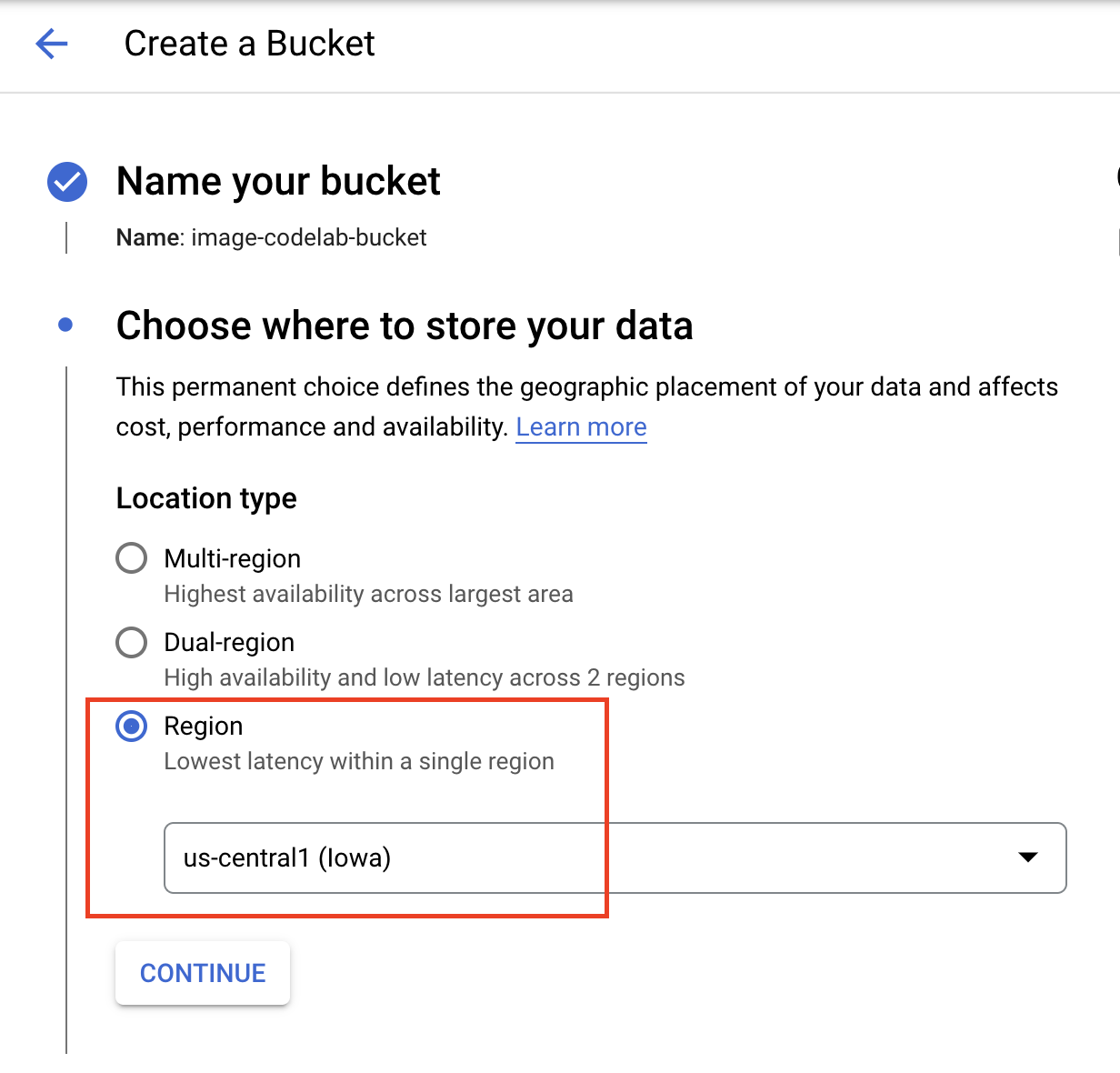Upload the TensorFlow hub model you downloaded to the bucket. Make sure you untar the file first.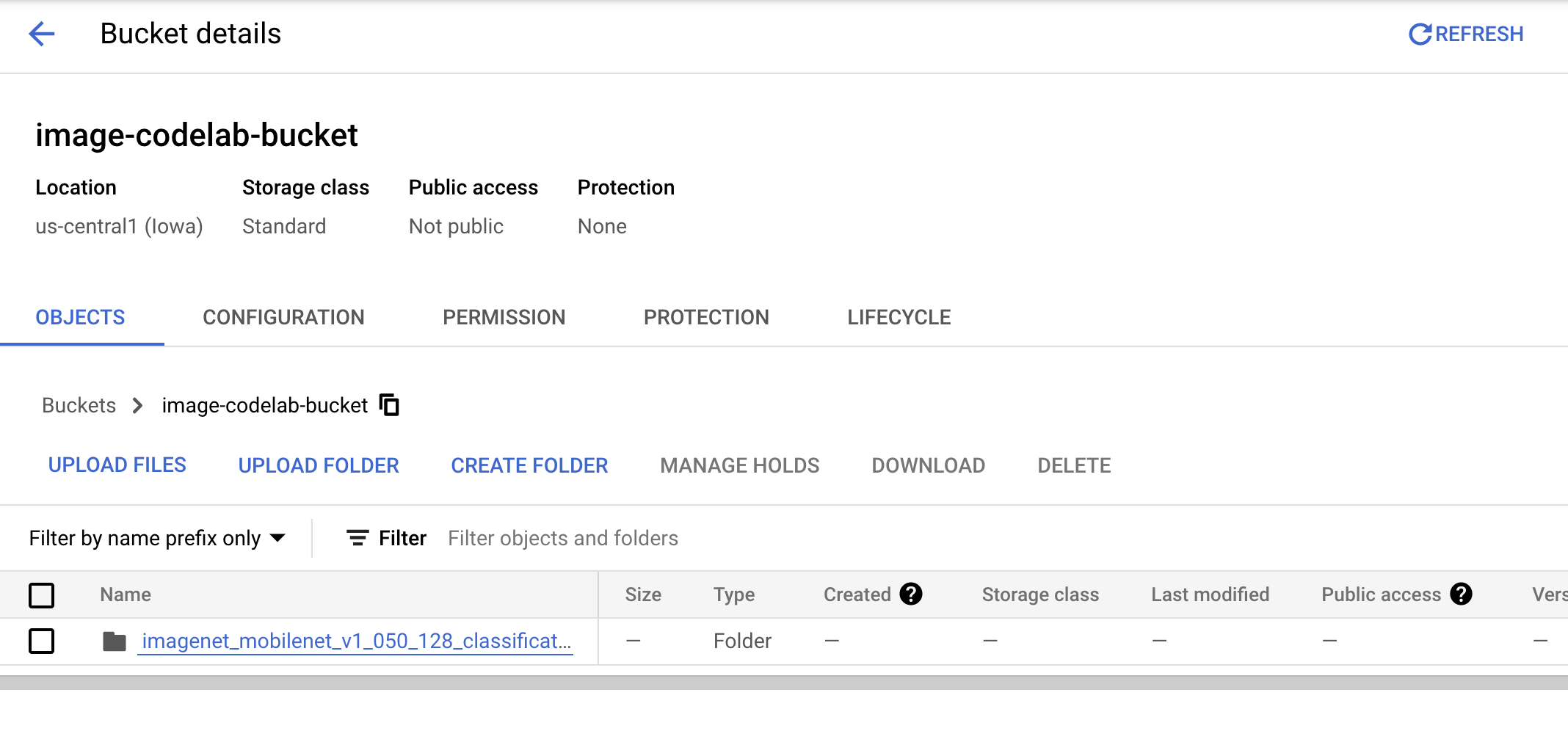Your bucket should look something like this:

``````imagenet_mobilenet_v1_050_128_classification_5/
saved_model.pb
variables/
variables.data-00000-of-00001
variables.index
``````

## Step 2: Import model to registry

Navigate to the Vertex AI Model registry section of the Cloud console.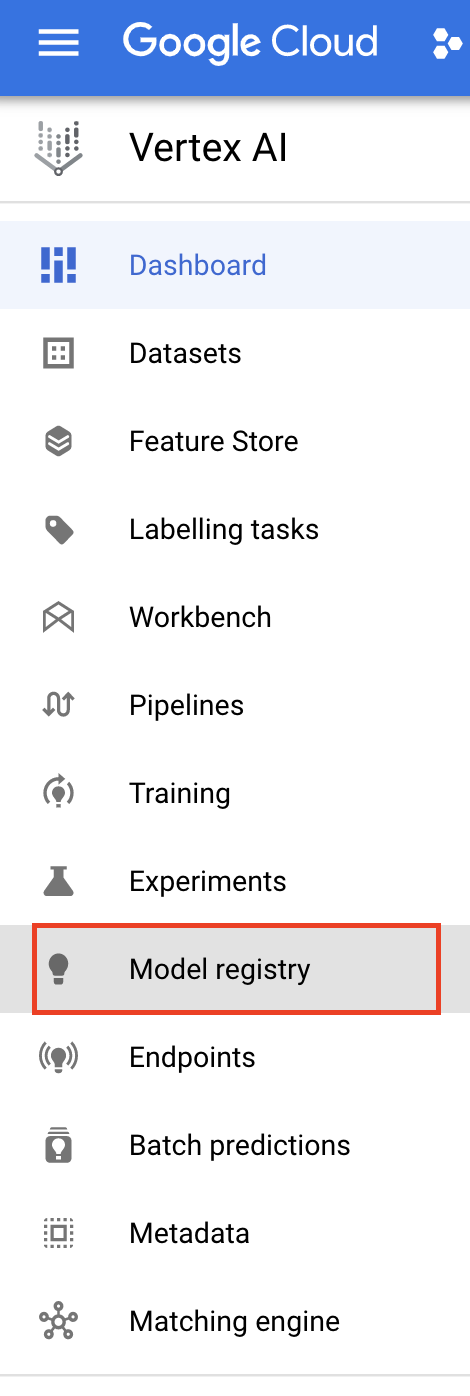Select IMPORT

Select Import as new model and then provide a name for your model.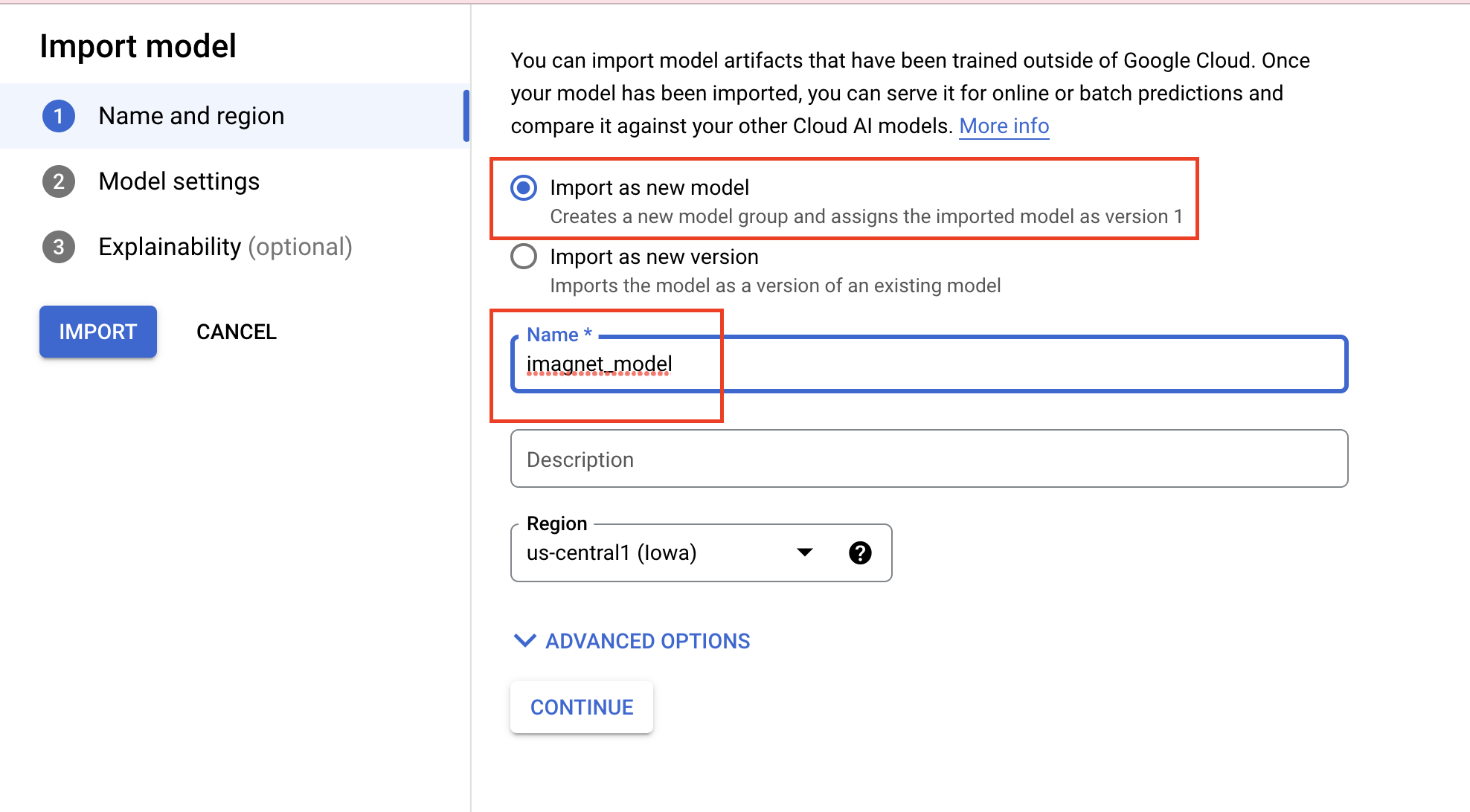Under Model settings specify the most recent pre-built TensorFlow container. Then, select the path in Cloud Storage where you stored the model artifacts.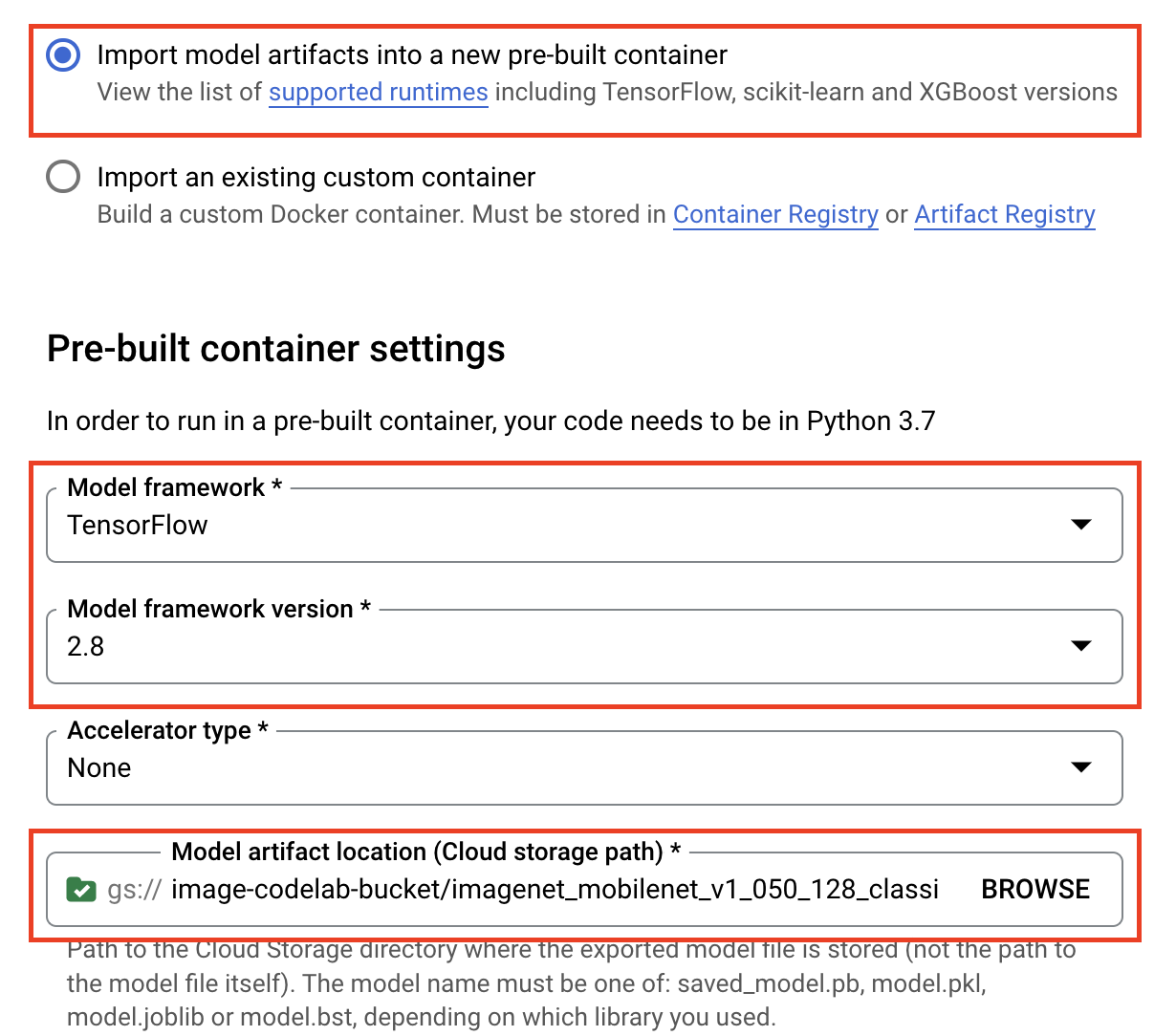You can skip the Explainability section.

Then select IMPORT

Once imported, you'll see your model in the model registry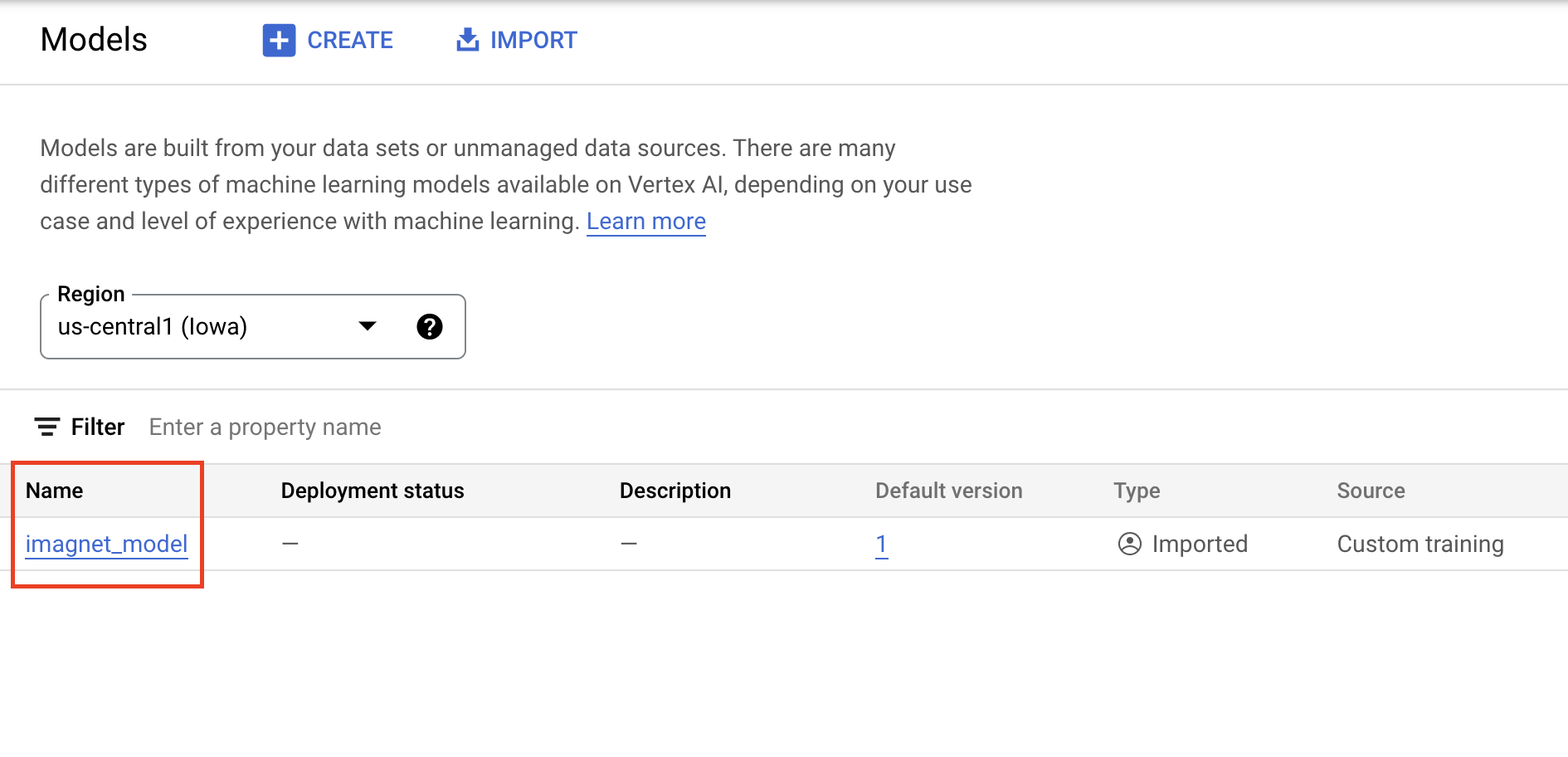## 6. Deploy model

From the Model registry, select the three dots on the right side of the model and click Deploy to endpoint.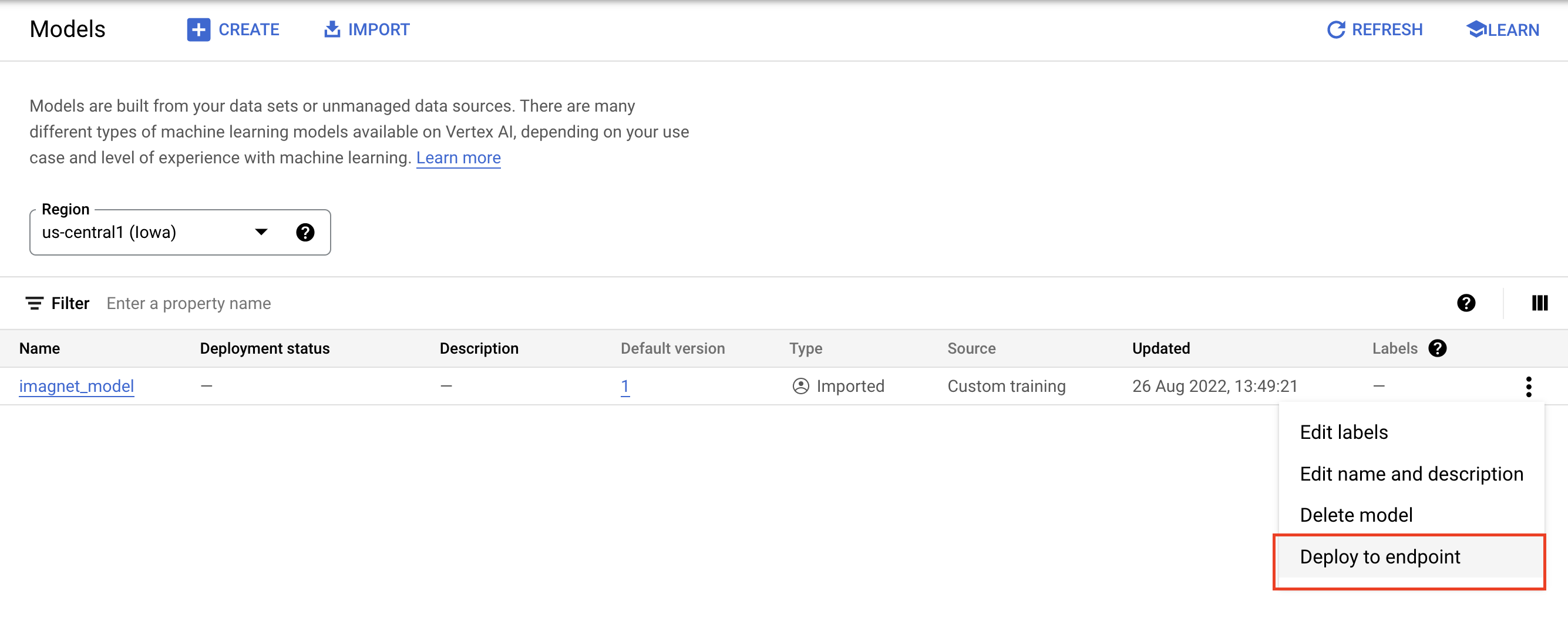Under Define your endpoint select create new endpoint and then give your endpoint a name.

Under Model settings, set the Maximum number of compute nodes to 1, and machine type to `n1-standard-2` and leave all other settings as is. Then click DEPLOY.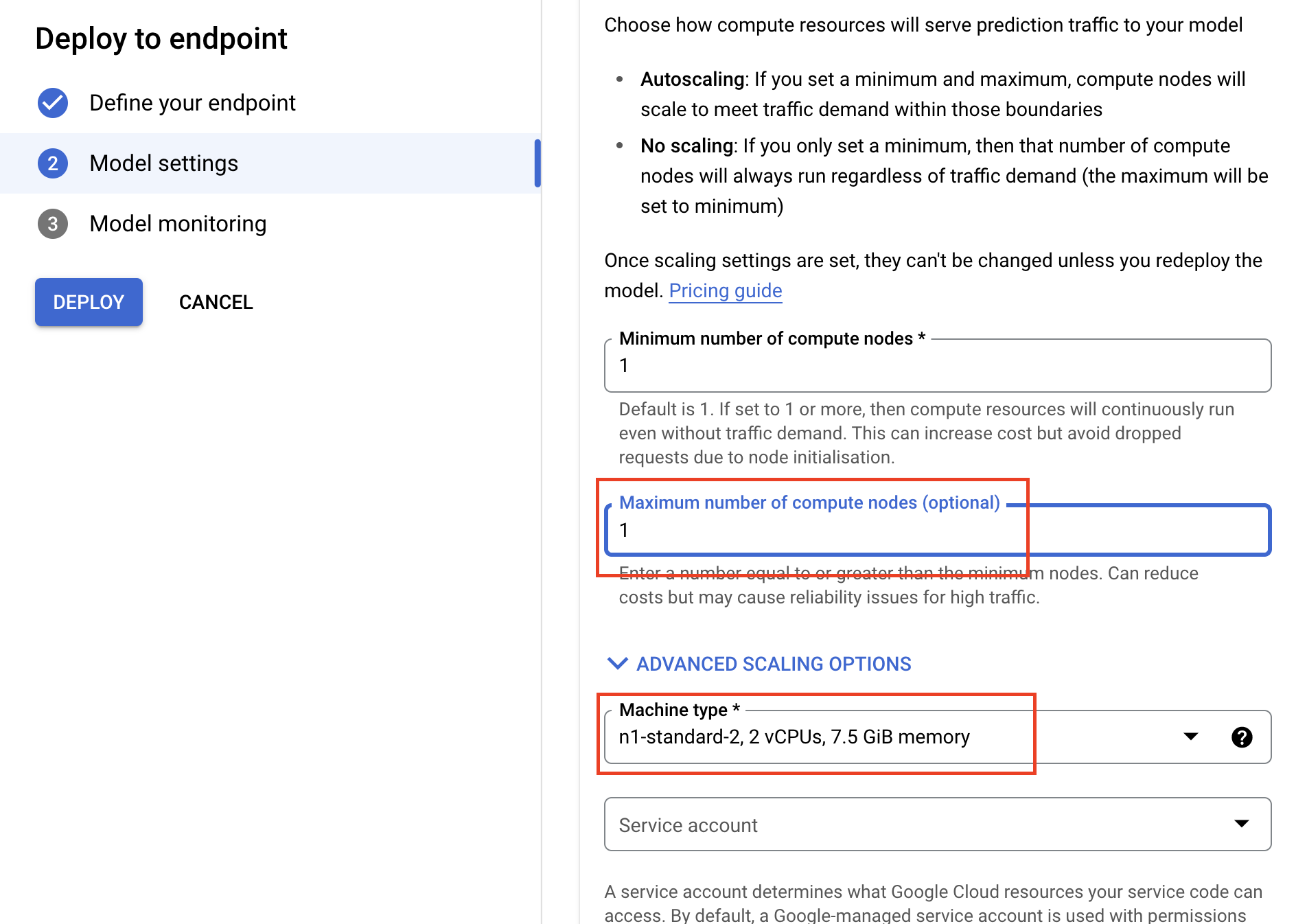When deployed, the deployment status will change to Deployed on Vertex AI.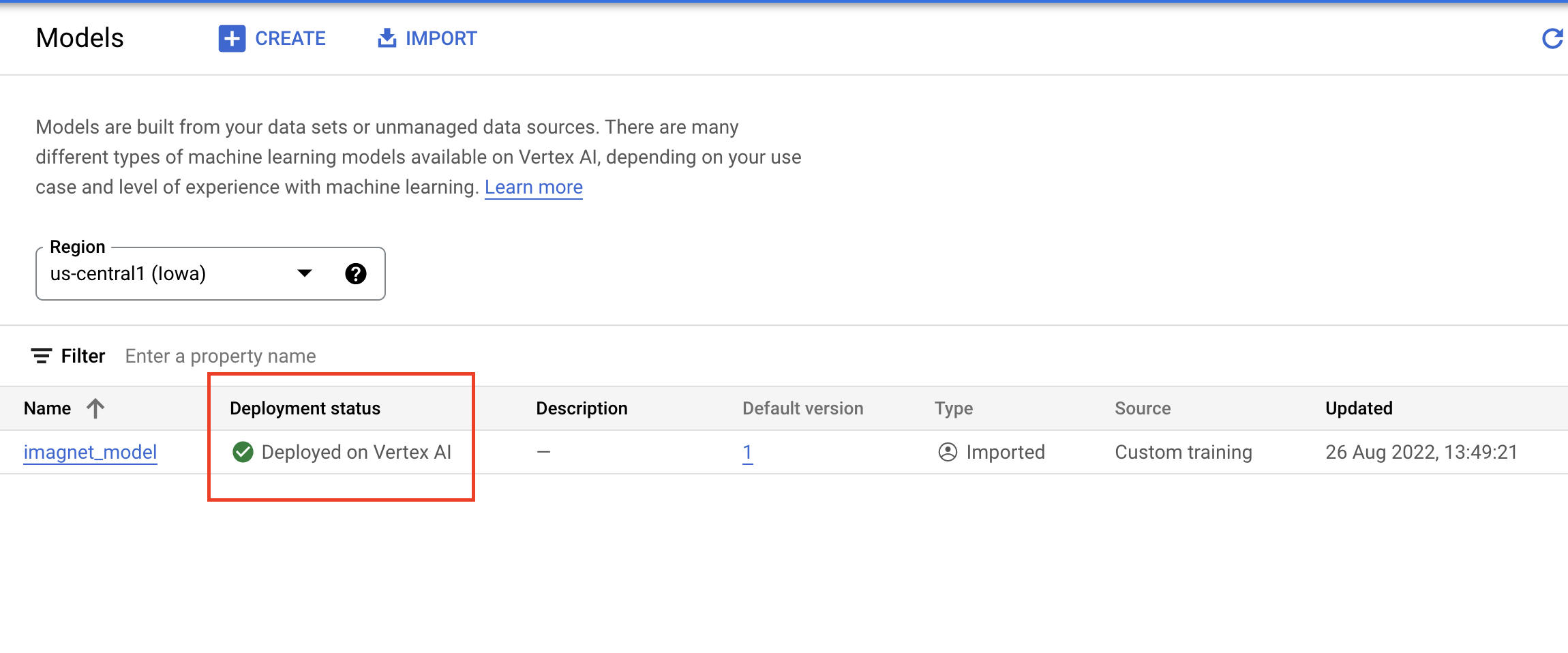## 7. Get predictions

Open up the Workbench notebook you created in the set up steps. From the launcher, create a new TensorFlow 2 notebook.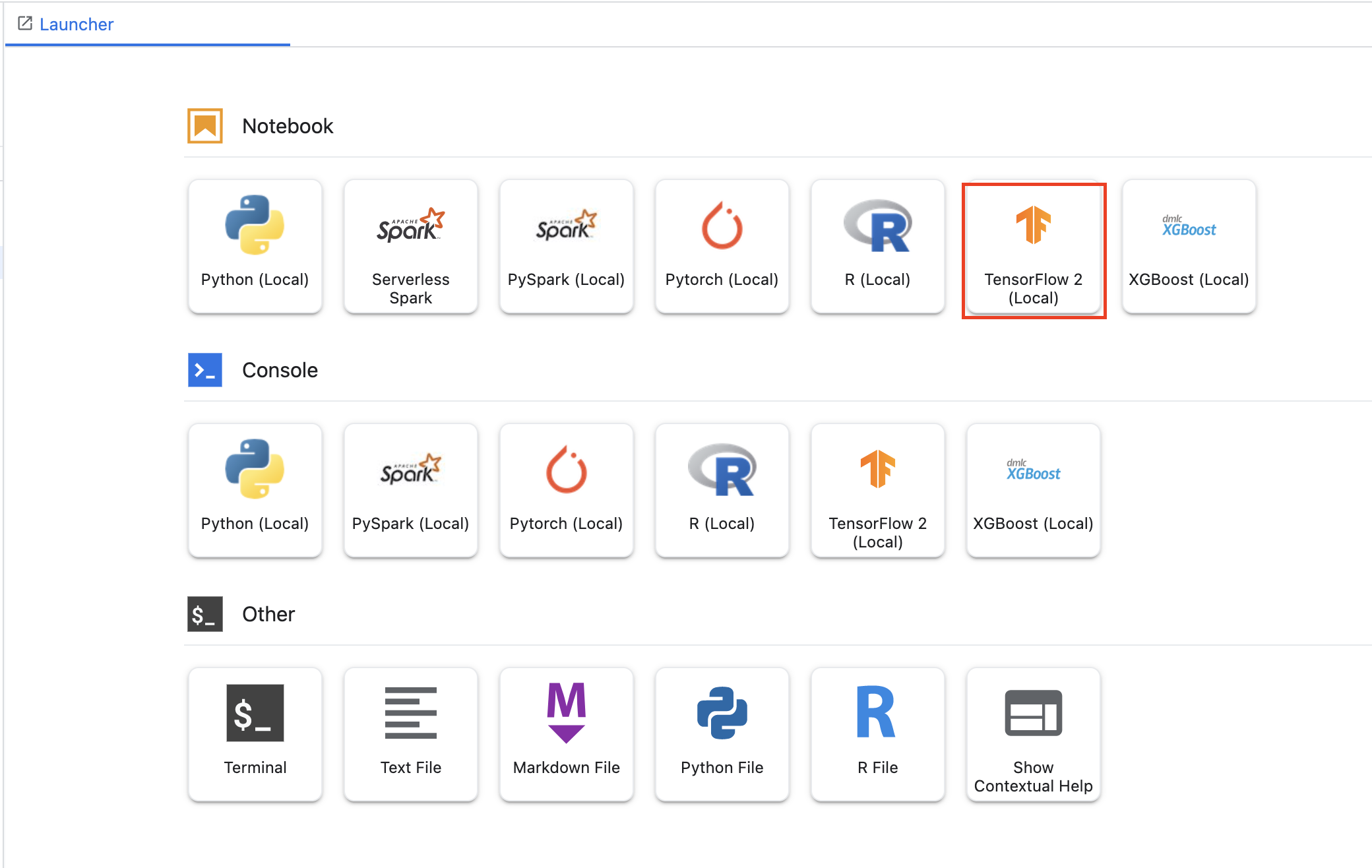Execute the following cell to import the necessary libraries

``````from google.cloud import aiplatform

import tensorflow as tf
import numpy as np
from PIL import Image
``````

The MobileNet model you downloaded from TensorFlow Hub was trained on the ImageNet dataset. The output of the MobileNet model is a number that corresponds to a class label in the ImageNet dataset. To translate that number into a string label, you'll need to download the image labels.

``````# Download image labels

``````

In order to hit the endpoint, you'll need to define the endpoint resource. Be sure to replace `{PROJECT_NUMBER}` and `{ENDPOINT_ID}`.

``````PROJECT_NUMBER = "{PROJECT_NUMBER}"
ENDPOINT_ID = "{ENDPOINT_ID}"

endpoint = aiplatform.Endpoint(
endpoint_name=f"projects/{PROJECT_NUMBER}/locations/us-central1/endpoints/{ENDPOINT_ID}")
``````

You can find your project number on the homepage of the console.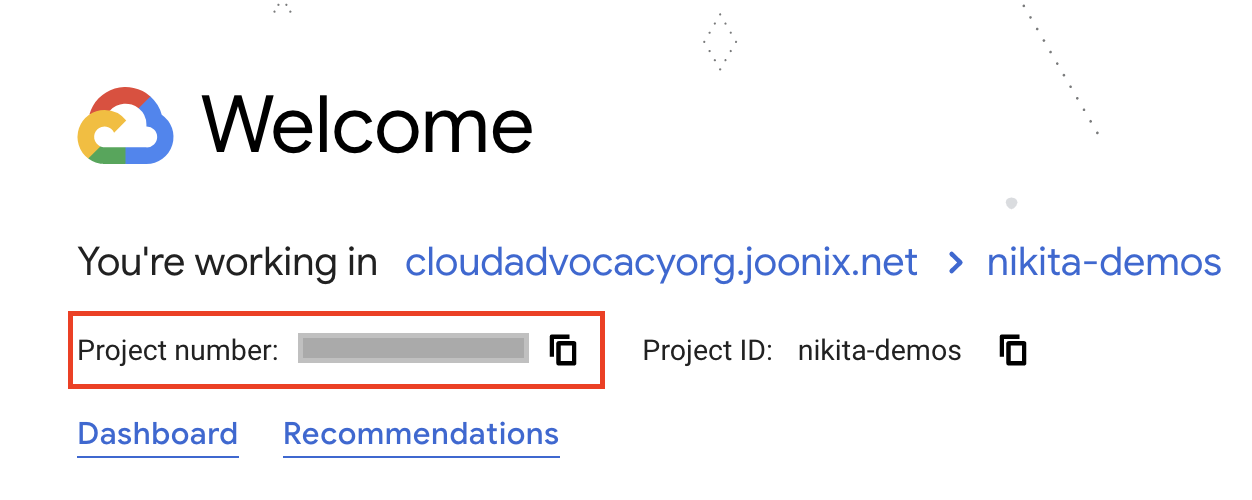And the endpoint ID on the Vertex AI Endpoints section.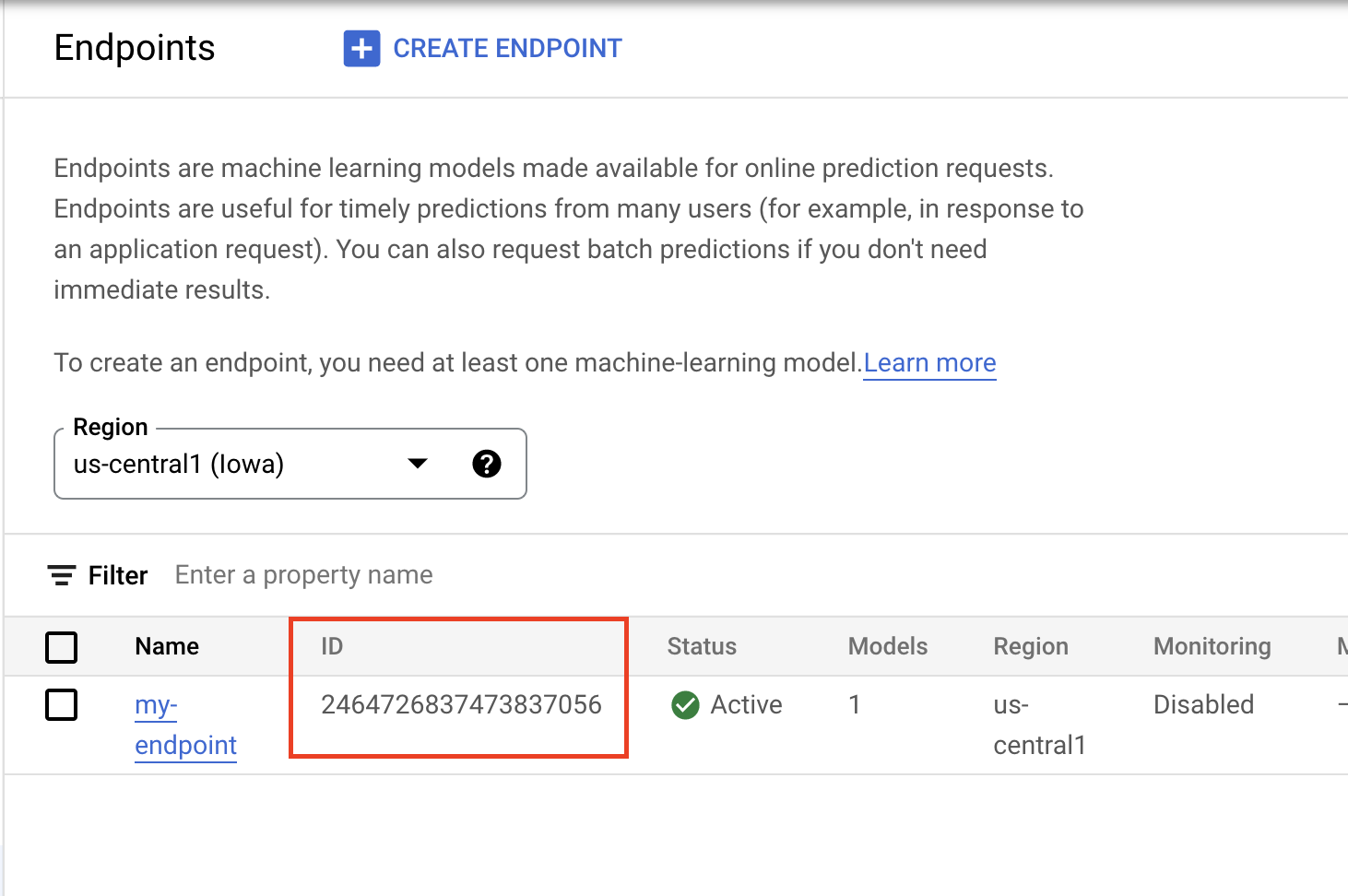Open the image with PIL. Then resize and scale by 255. Note that the image size expected by the model can be found on the model's TensorFlow hub page.

``````IMAGE_PATH = "test-image.jpg"
IMAGE_SIZE = (128, 128)

im = Image.open(IMAGE_PATH)
im = im.resize(IMAGE_SIZE
im = np.array(im)/255.0
``````

Next, convert the NumPy data to a list so it can be sent in the body of the http request.

``````x_test = im.astype(np.float32).tolist()
``````

Lastly, make a prediction call to the endpoint and then look up the corresponding string label.

``````# make prediction request
result = endpoint.predict(instances=[x_test]).predictions

# post process result
predicted_class = tf.math.argmax(result, axis=-1)
string_label = imagenet_labels[predicted_class]

print(f"label ID: {predicted_class}")
print(f"string label: {string_label}")
``````

## 8. [Optional] Use TF Serving to optimize predictions

For more realistic examples, you'll probably want to directly send the image itself to the endpoint, instead of loading it in NumPy first. This is more efficient but you'll have to modify the TensorFlow model's serving function. This modification is needed to convert the input data to the format your model expects.

## Step 1: Modify serving function

Open up a new TensorFlow notebook and import the necessary libraries.

``````from google.cloud import aiplatform

import tensorflow as tf
``````

Instead of downloading the saved model artifacts, this time you'll load the model into TensorFlow using `hub.KerasLayer`, which wraps a TensorFlow SavedModel as a Keras layer. To create the model, you can use the Keras Sequential API with the downloaded TF Hub model as a layer, and specify the input shape to the model.

``````tfhub_model = tf.keras.Sequential(
)
tfhub_model.build([None, 128, 128, 3])
``````

Define the URI to the bucket you created earlier.

``````BUCKET_URI = "gs://{YOUR_BUCKET}"
MODEL_DIR = BUCKET_URI + "/bytes_model"
``````

When you send a request to an online prediction server, the request is received by a HTTP server. The HTTP server extracts the prediction request from the HTTP request content body. The extracted prediction request is forwarded to the serving function. For the Vertex AI pre-built prediction containers, the request content is passed to the serving function as a `tf.string`.

To pass images to the prediction service, you will need to encode the compressed image bytes into base 64, which makes the content safe from modification while transmitting binary data over the network.

Since the deployed model expects input data as raw (uncompressed) bytes, you need to ensure that the base 64 encoded data gets converted back to raw bytes (eg JPEG), and then preprocessed to match the model input requirements, before it is passed as input to the deployed model.

To resolve this, you define a serving function (`serving_fn`) and attach it to the model as a preprocessing step. You add a `@tf.function` decorator so the serving function is fused to the underlying model (instead of upstream on a CPU).

``````CONCRETE_INPUT = "numpy_inputs"

def _preprocess(bytes_input):
decoded = tf.io.decode_jpeg(bytes_input, channels=3)
decoded = tf.image.convert_image_dtype(decoded, tf.float32)
resized = tf.image.resize(decoded, size=(128, 128))
return resized

@tf.function(input_signature=[tf.TensorSpec([None], tf.string)])
def preprocess_fn(bytes_inputs):
decoded_images = tf.map_fn(
_preprocess, bytes_inputs, dtype=tf.float32, back_prop=False
)
return {
CONCRETE_INPUT: decoded_images
}  # User needs to make sure the key matches model's input

@tf.function(input_signature=[tf.TensorSpec([None], tf.string)])
def serving_fn(bytes_inputs):
images = preprocess_fn(bytes_inputs)
prob = m_call(**images)
return prob

m_call = tf.function(tfhub_model.call).get_concrete_function(
[tf.TensorSpec(shape=[None, 128, 128, 3], dtype=tf.float32, name=CONCRETE_INPUT)]
)

tf.saved_model.save(tfhub_model, MODEL_DIR, signatures={"serving_default": serving_fn})
``````

When you send data for prediction as an HTTP request packet, the image data is base64 encoded, but the TensorFlow model takes numpy input. Your serving function will do the conversion from base64 to a numpy array.

When making a prediction request, you need to route the request to the serving function instead of the model, so you need to know the input layer name of the serving function. We can get this name from the serving function signature.

``````loaded = tf.saved_model.load(MODEL_DIR)

serving_input = list(
)
print("Serving function input name:", serving_input)
``````

## Step 2: Import to registry and deploy

In the previous sections you saw how to import a model to the Vertex AI Model Registry via the UI. In this section you'll see an alternate way using the SDK instead. Note that you can still use the UI here instead if you prefer.

``````model = aiplatform.Model.upload(
display_name="optimized-model",
artifact_uri=MODEL_DIR,
serving_container_image_uri="us-docker.pkg.dev/vertex-ai/prediction/tf2-cpu.2-8:latest",
)

print(model)
``````

You can also deploy the model using the SDK, instead of the UI.

``````endpoint = model.deploy(
deployed_model_display_name='my-bytes-endpoint',
traffic_split={"0": 100},
machine_type="n1-standard-4",
accelerator_count=0,
min_replica_count=1,
max_replica_count=1,
)
``````

## Step 3: Test model

Now you can test the endpoint. Because we modified the serving function, this time you can send the image directly (base64 encoded) in the request instead of loading the image into NumPy first. This will also allow you to send larger images without hitting the Vertex AI Predictions size limit.

``````import numpy as np
``````

Base64 encode the image.

``````import base64

with open("test-image.jpg", "rb") as f:
b64str = base64.b64encode(data).decode("utf-8")
``````

Make a prediction call, specifying the input layer name of the serving function which we defined in the `serving_input` variable earlier.

``````instances = [{serving_input: {"b64": b64str}}]

# Make request
result = endpoint.predict(instances=instances).predictions

# Convert image class to string label
predicted_class = tf.math.argmax(result, axis=-1)
string_label = imagenet_labels[predicted_class]

print(f"label ID: {predicted_class}")
print(f"string label: {string_label}")
``````

🎉 Congratulations! 🎉

You've learned how to use Vertex AI to:

• Host and deploy a pre-trained model